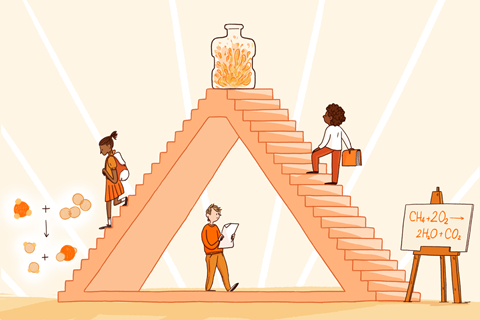Help students make links between macroscopic, symbolic and sub-microscopic levels

As science teachers it’s our job to make science accessible. This presents its own challenges. Science happens at a visible level, but the explanations are often unseen or on an abstract level. As science teachers we have to bridge the gap between the seen and unseen, which we can do through modelling.### Johnstone’s triangle

Alex Johnstone suggested that chemistry knowledge is understood at three fundamental ‘levels’ or ‘representations’: the macroscopic, the symbolic and the sub-microscopic.

The macroscopic is the ‘seen’ level, eg practical investigation or observations, which can be described. The symbolic level is the unseen: how we represent the macroscopic through word or symbol equations, mathematical formula, graphs and diagrams. Finally the sub-microscopic is also unseen, at the atomic level, and includes explanatory models. For students to gain a deeper awareness of a topic, they need to understand it at all three levels.

Download a worksheet template for introducing the triangle as MS Word or pdf

### Using the triangle

You can use the triangle in a single lesson, or as part of a topic, to develop understanding between the levels. It can also help reduce information overload.

• #### I try – introducing the triangle

Why, why, why. It’s not only important to share the structure of the triangle with students prior to use but also tell them why you want them to use the triangle. It will also help gain student buy-in. You could share an example of a completed triangle for a concept as you introduce each level. I shared a rates of reaction triangle with students before we used it for combustion. Students can see how the concept breaks down, with examples of what each level looks like.

• #### Let’s try

After introducing the triangle, share macroscopic examples with students first; I shared methane bubbles and the Whoosh bottle. After recording our observations, we discussed why these were macro, reinforcing that level. Then move on to the second level, the symbolic, for example using a word equation. You can use Molymods to explain what happens at the atomic level, followed by symbol equations. Again, we discussed where each should go on their triangle and why.

• #### You try

After completing the macro and symbolic parts of the triangle, it’s their turn. With my students, I introduced incomplete combustion at the centre of the triangle, and I asked: how is complete combustion different to incomplete combustion? We followed up further practical work with physical modelling and word equations. Students had to decide where these fit on their triangles.

### 7 simple rules to boost science teaching

Click to expand and explore the rules

Use practical work purposefully and as part of a learning sequence

Develop scientific vocabulary and support pupils to read and write about science

Use structured feedback to move on pupils’ thinking

### Practical work and the triangle

Students can lack macroscopic experiences in chemistry so you could introduce a concept with a prepared triangle. Working as a team, students get macroscopic variety and you can also ensure that the links between levels are clear. For example, the macro and symbolic can be the sole focus for core practical investigations at GCSE. Students can photograph the practical (macroscopic level) as visual reminders. They can relate to the symbolic through apparatus diagrams, formula and/or word equations. Initially focusing on just two levels can avoid overwhelming students, and helps you to differentiate for groups. You can build the level of challenge to include the sub-microscopic.

### Final thoughts

Johnstone’s triangle has sparked further research and discussion in chemistry education particularly around how it has been interpreted and taught. Regardless, it draws attention to how scientific knowledge is understood at more than one representation or level, enabling students to make explicit links between these levels.

This article is part of the series 7 simple rules for science teaching, developed in response to the EEF’s Improving secondary science guidance. It supports rule 3a, Use models to help pupils develop a deeper understanding of scientific concepts.